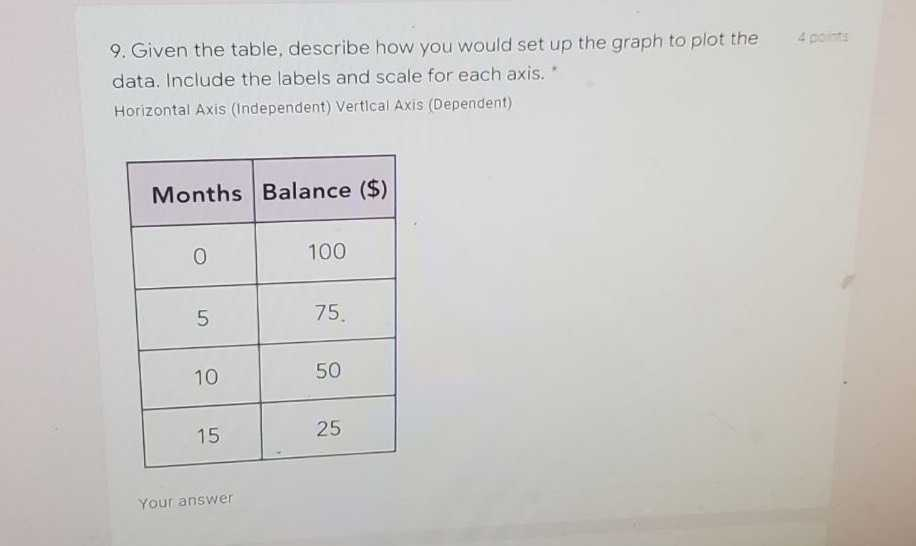### ¿Todavía tienes preguntas de matemáticas?

Pregunte a nuestros tutores expertos
Algebra
Pregunta9 Given the table, describe how you would set up the graph to plot the data. Include the labels and scale for each axis. Horizontal Axis (Independent) Vertical Axis (Dependent)

Slope Intercept Form: $$y = - 5x + 100$$